笔试出了一个简单的排序题。他是想考察啥的

meluo 发布于 2015/03/20 19:26

【福利】CTO直接拍板说好用的SDK全在这里了！0元配齐，全部免费！>>>0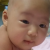00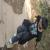0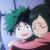0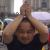“莫用程序思维去揣度人心”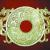0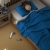public class Test {
public static void main(String[] args) {
Do<Integer> d = new Do<Integer>(10, 5);
System.out.println("***********************");
System.out.println(d.todo(new Multiply()));
}
}

class Multiply implements Plugin<Integer> {
@Override
public Integer todo(Integer t1, Integer t2) {
return t1 * t2;
}
}

class Add implements Plugin<Integer> {
@Override
public Integer todo(Integer t1, Integer t2) {
return t1 + t2;
}
}

class Do<T> {
private T x;
private T y;

public Do(T x, T y) {
this.x = x;
this.y = y;
}

public T todo(Plugin<T> plugin) {
return plugin.todo(this.x, this.y);
}
}

interface Plugin<T> {
public T todo(T t1, T t2);
}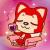0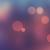public enum Calculator {

@Override
public int exec(int a, int b) {
return a + b;
}

},

SUB("-") {
public int exec(int a, int b) {
return a - b;
}
};

String value;

private Calculator(String value) {
this.value = value;
}

public String getValue() {
return this.value;

}

public abstract int exec(int a, int b);

}

0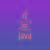引用来自“成为朝拜者”的评论

public enum Calculator {

@Override
public int exec(int a, int b) {
return a + b;
}

},

SUB("-") {
public int exec(int a, int b) {
return a - b;
}
};

String value;

private Calculator(String value) {
this.value = value;
}

public String getValue() {
return this.value;

}

public abstract int exec(int a, int b);

}

10分能给你2分就不错了，1. 乘除运算没得 2. 运算溢出没考虑 3. 若把ADD、SUB看作是操作符，真心不知道为什么要一个value成员，即使要换个名字可好0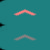0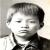引用来自“IMU”的评论